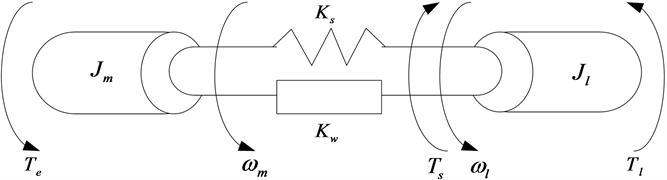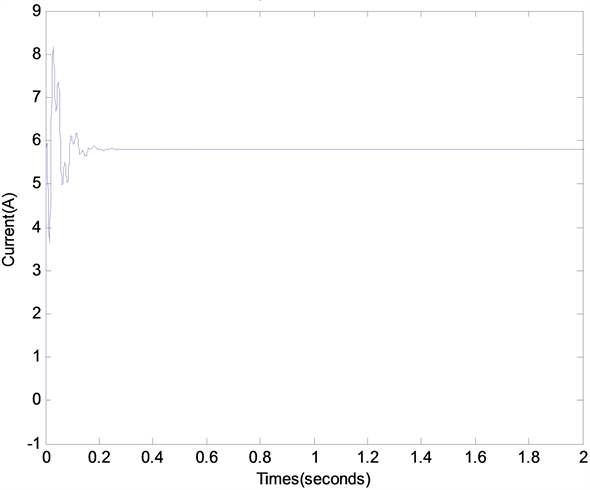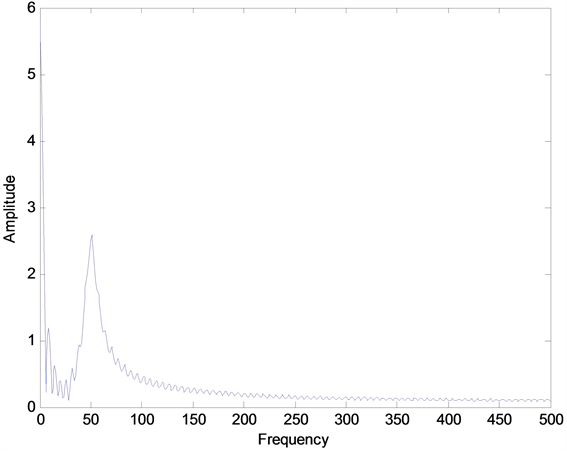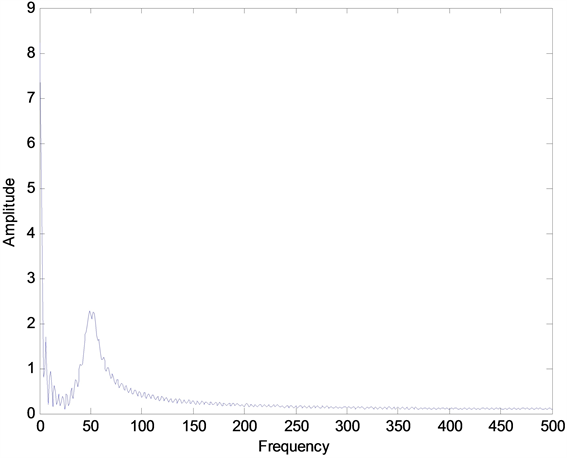﻿ 基于机器学习算法的谐振点漂移的伺服系统最优谐振抑制

# 基于机器学习算法的谐振点漂移的伺服系统最优谐振抑制 Optimal Resonance Suppression of Servo System with Resonance Point Drift Based on Machine Learning Algorithm

Abstract: In order to suppress the resonance of the servo system when the resonance point drifts, a notch filter is connected in the servo system to suppress the mechanical resonance of the two mass system. Firstly, the mathematical model of two mass system is established, the resonance mechanism is analyzed, and the causes of mechanical resonance are described; Secondly, the online search of drift resonance point is carried out by using the trisection method; Thirdly, the principle of notch filter is analyzed, and the width and depth parameters of notch filter are determined by simulated annealing algorithm; Then, on the basis of determining the optimal parameters, the robustness of the system is analyzed; Finally, based on the determination of the optimal parameters, experiments and system robustness analysis are carried out. The experimental results show that this method can not only quickly search the drift resonance point, effectively suppress the resonance and maintain the stability of the system, but also avoid the defect of time-consuming manual adjustment of parameters, and can accurately and quickly suppress the drift resonance point.

1. 引言

1) 利用三分法在线搜索谐振频率的效率高，搜索速度是现有文献中提出算法的搜索速度的10至20倍；

2) 利用机器学习算法中的模拟退火算法求解到陷波滤波器的最优参数，可以达到最优谐振抑制的目的；

3) 本文研究的谐振抑制方法既能抑制机械谐振，又能保持系统的稳定性；

4) 本文提出的最优谐振抑制方法可以快速、准确抑制谐振，避免手动调节参数耗时长的缺陷，提高谐振抑制的效率。

2. 二质量系统模型建立Figure 1. Simplified model of two-mass system

$\left\{\begin{array}{l}{J}_{m}\frac{\text{d}{\omega }_{m}}{\text{d}t}={T}_{e}-{T}_{s}\\ {J}_{l}\frac{\text{d}{\omega }_{l}}{\text{d}t}={T}_{s}-{T}_{l}\\ {T}_{s}={K}_{s}\left({\theta }_{m}-{\theta }_{l}\right)+{K}_{W}\left({\omega }_{m}-{\omega }_{l}\right)\\ {\stackrel{˙}{\theta }}_{m}={\omega }_{m}\\ {\stackrel{˙}{\theta }}_{l}={\omega }_{l}\end{array}$ (1)

$G\left(s\right)=\frac{1}{{J}_{m}s}×{G}_{r}\left(s\right)=\frac{1}{{J}_{m}s}\frac{{s}^{2}+2p{\xi }_{r}{\omega }_{n}s+p{\omega }_{n}^{2}}{{s}^{2}+2{\xi }_{r}{\omega }_{n}s+{\omega }_{n}^{2}}$ (2)

${G}_{r}\left(s\right)=\frac{{s}^{2}+2p{\xi }_{r}{\omega }_{n}s+p{\omega }_{n}^{2}}{{s}^{2}+2{\xi }_{r}{\omega }_{n}s+{\omega }_{n}^{2}}$

$\left\{\begin{array}{l}{\xi }_{r}=\sqrt{\frac{\left({J}_{m}+{J}_{l}\right){K}_{W}^{2}}{4{K}_{s}{J}_{m}{J}_{l}}}\\ {\omega }_{n}=\sqrt{\frac{{K}_{s}\left({J}_{m}+{J}_{l}\right)}{{J}_{m}{J}_{l}}}\\ {\omega }_{an}=\sqrt{\frac{{K}_{s}}{{J}_{l}}}\\ p=\frac{{J}_{m}}{{J}_{m}+{J}_{l}}\end{array}$ (3)

3. 漂移谐振点的在线搜索

1) 如果 $h\left({x}_{1}\right)>h\left({x}_{2}\right)$，则谐振点位于区间 $\left[a,{x}_{2}\right]$，计算区间长度l；

2) 如果 $h\left({x}_{1}\right)=h\left({x}_{2}\right)$，则谐振点位于区间 $\left[{x}_{1},{x}_{2}\right]$，计算区间长度l；

3) 如果 $h\left({x}_{1}\right)，则谐振点位于区间 $\left[{x}_{1},b\right]$，计算区间长度l。

4. 陷波滤波器最优谐振抑制

4.1. 陷波滤波器原理

${G}_{N}\left(s\right)=\frac{{s}^{2}+2\pi \xi ks+{\omega }_{0}^{2}}{{s}^{2}+2\pi ks+{\omega }_{0}^{2}}$ (4)

${G}_{0}\left(s\right)={G}_{r}\left(s\right){G}_{N}\left(s\right)$ (5)

4.2. 陷波滤波器的参数确定

${G}_{o}\left(s\right)={G}_{r}\left(s\right){G}_{N}\left(s\right)=\frac{{s}^{2}+2p{\xi }_{r}{\omega }_{n}s+p{\omega }_{n}^{2}}{{s}^{2}+2{\xi }_{r}{\omega }_{n}s+{\omega }_{n}^{2}}\cdot \frac{{s}^{2}+2\pi \xi ks+{\omega }_{0}^{2}}{{s}^{2}+2\pi ks+{\omega }_{0}^{2}}$ (6)

$s=j\omega$，则级联陷波器在任意频率处的幅值为：

${H}_{0}\left(\omega \right)=|{G}_{0}\left(j\omega \right)|=\sqrt{\frac{{\left(j\omega \right)}^{2}+2p{\xi }_{r}{\omega }_{n}\left(j\omega \right)+p{\omega }_{n}^{2}}{{\left(j\omega \right)}^{2}+2{\xi }_{r}{\omega }_{n}\left(j\omega \right)+{\omega }_{n}^{2}}}\sqrt{\frac{{\left(j\omega \right)}^{2}+2\pi \xi k\left(j\omega \right)+{\omega }_{0}^{2}}{{\left(j\omega \right)}^{2}+2\pi k\left(j\omega \right)+{\omega }_{0}^{2}}}$ (7)Figure 2. Bode diagram of a two mass system with a given reference threshold

$\underset{{\omega }_{i}\in \left[{\omega }_{1},{\omega }_{2}\right]}{{H}_{0}\left({\omega }_{i}\right)}\le {H}_{th}$ (8)

$\varphi =\text{arctan}\frac{2\pi \left(\xi -1\right)k{\omega }_{c}\left({\omega }_{0}^{2}-{\omega }_{c}^{2}\right)}{4{\pi }^{2}\xi k{\omega }_{c}^{2}+{\left({\omega }_{0}^{2}-{\omega }_{c}^{2}\right)}^{2}}$ (9)

$\underset{\underset{{\omega }_{i}\in \left[{\omega }_{1},{\omega }_{2}\right]}{{H}_{0}\left({\omega }_{i}\right)\le {H}_{th}}}{\mathrm{min}}\varphi =\mathrm{arctan}\frac{2\pi \left(\xi -1\right)k{\omega }_{c}\left({\omega }_{0}^{2}-{\omega }_{c}^{2}\right)}{4{\pi }^{2}\xi k{\omega }_{c}^{2}+{\left({\omega }_{0}^{2}-{\omega }_{c}^{2}\right)}^{2}}$ (10)

Step 1 选取最大迭代次数 ${K}_{\mathrm{max}}$，可行域内任意收敛方向 ${v}_{r}$ 和最优收敛方向 ${v}_{b}$，以及可行域内任意初始状态 ${x}_{0}=\left({\xi }_{0},{k}_{0}\right)$

Step 2 令 $T\left(i\right)=1-i/\left({K}_{\mathrm{max}}+1\right)$，则T单调递减趋于0，随机选择正参数 $\lambda$，令 $v\left(i\right)=T\left(i\right){v}_{r}+\left(1-T\right){v}_{b}$${x}_{i}={x}_{0}+\lambda \left(i\right)v\left(i\right)$

Step 3 计算相角裕度 $\phi \left({x}_{0}\right)$$\phi \left({x}_{i}\right)$ 并进行比较，如果满足 $\phi \left({x}_{0}\right)>\phi \left({x}_{i}\right)$，就将 ${x}_{i}$ 接收为新的初始状态；否则以概率 $p=\mathrm{exp}\left\{-\left(f\left({x}_{i}\right)-f\left({x}_{0}\right)\right)/T\left(i\right)\right\}$ 接收 ${x}_{i}$ 为新的状态；

Step 4 重复Step 3，直至得到对可行域内任意的 ${x}_{j}$，都有 $\phi \left({x}_{j}\right)<\phi \left({x}^{\ast }\right)$ 或达到最大迭代次数时的相角裕度最小值所对应的 ${x}^{\ast }$，则得到的最优解为 ${x}^{\ast }$

5. 实验

5.1. 谐振抑制实验Table 1. Parameters of permanent magnet synchronous motorFigure 3. Experimental setupFigure 4. Current responseFigure 5. Speed response(a)(b)

Figure 6. Spectrum; (a) Spectrum before suppression; (b) Spectrum after suppression

5.2. 鲁棒性分析Figure 7. Robustness analysis results

6. 总结

 李云松, 陈小安. 精密传动系统伺服驱动机械谐振灵敏度分析[J]. 机械传动, 2017, 41(4): 24-27.

 Szabat, K. and Orlowska Kowalska, T. (2007) Vibration Suppression in a Two-Mass Drive System Using PI Speed Controller and Additional Feedbacks: Comparative Study. IEEE Trans on Industrial Electronics, 54, 1193-1206.
https://doi.org/10.1109/TIE.2007.892608

 Orlowska Kowalska, T. and Kaminski, M. (2009) Effectiveness of Saliency Based Methods in Optimization of Neural State Estimators of the Drive System with Elastic Couplings. IEEE Trans on Industrial Electronics, 56, 4043-4051.
https://doi.org/10.1109/TIE.2009.2027250

 Katsura, S. and Ohnishi, K. (2007) Force Servoing by Flexible Manipulator Based on Resonance Ratio Control. IEEE Trans on Industrial Electronics, 54, 539-547.
https://doi.org/10.1109/TIE.2006.888805

 Muszynski, R. and Deskur, J. (2010) Damping of Torsional Vibra-tions in High-Dynamic Industrial Drives. IEEE Trans on Industrial Electronics, 57, 544-552.
https://doi.org/10.1109/TIE.2009.2036034

 Hace, A., Jezernik, K. and Abanovic, A. (2007) SMC with Dis-turbance Observer for a Linear Belt Drive. IEEE Trans on Industrial Electronics, 54, 3402-3412.
https://doi.org/10.1109/TIE.2007.906130

 Peter, K., Schling, I. and Orlik, B. (2003) Robust Output- Feedback H∞-Control with a Nonlinear Observer for a Two- Mass System. IEEE Trans on Industry Applications, 39, 637-644.
https://doi.org/10.1109/TIA.2003.811788

 Cychowski, M., Szabat, K. and Orlowska Kowalska, T. (2009) Constrained Model Predictive Control of the Drive System with Mechanical Elasticity. IEEE Trans on Industry Applica-tions, 56, 1963-1973.
https://doi.org/10.1109/TIE.2009.2015753

 Zhang, G. (2000) Speed Control of Two-Inertia System by PI/PID Control. IEEE Transactions on Industrial Electronics, 47, 603-609.
https://doi.org/10.1109/41.847901

 杨明, 王璨, 徐殿国. 基于轴矩限幅控制的机械谐振抑制技术[J]. 电机与控制学报, 2015, 19(4): 58-64.

 熊琰, 李叶松. 基于频率特性搜索的伺服系统谐振控制[J]. 电气传动, 2015, 45(2): 39-44+80.

 周凤岐, 周军, 郭建国. 现代控制理论基础[M]. 西安: 西北工业大学出版社, 2011.

 Cai, L.-H., Gao, H.-B., Zhang, S.-M. and Ma, Q.-K. (2011) Research on Method of Eliminating Accurately Mechanical Resonance Frequency of the Theodolite by complex Notch Filter. 2011 International Conference on System Science, Engineering Design and Manufacturing Information, Guiyang, 22-23 October 2011, 60-63.
https://doi.org/10.1109/ICSSEM.2011.6081329

 Kwan, T. and Martin, K. (1989) Adaptive Detection and En-hancement of Multiple Sinusoids Using a Cascade IIR Filter. IEEE Transactions on Circuits and Systems, 36, 937-947.
https://doi.org/10.1109/31.31329

 王昱忠. 伺服系统机械谐振抑制方法的研究与实现[D]: [硕士学位论文]. 沈阳: 中国科学院大学(中国科学院沈阳计算技术研究所), 2018.

 杨明, 郝亮, 徐殿国. 基于自适应陷波滤波器的在线机械谐振抑制[J]. 哈尔滨工业大学学报, 2014, 46(4): 63-69.

 康健. 伺服系统机械谐振在线检测及抑制方法研究[D]: [硕士学位论文]. 哈尔滨: 哈尔滨工业大学, 2012.

 Information Technology (2020) Research Data from Sharif University of Technology Update Understanding of Information Technology (Frequency Da-ta-Based Procedure to Adjust Gain and Phase Margins and Guarantee the Uniqueness of Crossover Frequencies). p. 507

 陈梦沂. 模拟退火算法的改进[J]. 通化师范学院学报, 2017, 38(10): 41-44.

 姚新, 陈国良. 模拟退火算法及其应用[J]. 计算机研究与发展, 1990(7): 1-6.

 谢云. 模拟退火算法的原理及实现[J]. 高等学校计算数学学报, 1999(3): 212-218.

 王昱忠, 何平, 王志成, 韩旭. 基于自适应陷波器的伺服系统谐振抑制[J]. 组合机床与自动化加工技术, 2019(1): 68-71.

Top